# GSEB Solutions Class 12 Maths Chapter 4 Determinants Ex 4.6

Gujarat Board GSEB Textbook Solutions Class 12 Maths Chapter 4 Determinants Ex 4.6 Textbook Questions and Answers.

## Gujarat Board Textbook Solutions Class 12 Maths Chapter 4 Determinants Ex 4.6Question 1.
x + 2y = 2
2x + 3y = 3
Solution:
x + 2y = 2
2x + 3y = 3
Let A = $$\left[\begin{array}{ll} 1 & 2 \\ 2 & 3 \end{array}\right]$$
∴ |A| = $$\left|\begin{array}{ll} 1 & 2 \\ 2 & 3 \end{array}\right|$$ = 3 – 4 = – 1
So, |A| ≠ 0.
∴ System of equations has a unique solution.
Hence, the system of equations is consistent.

Question 2.
2x – y = 5
x + y = 4
Solution:
2x – y = 5
x + y = 4
Let A = $$\left[\begin{array}{ll} 2 & -1 \\ 1 & 1 \end{array}\right]$$
∴ |A| = $$\left|\begin{array}{ll} 2 & -1 \\ 1 & 1 \end{array}\right|$$ = 2 + 1 = 3 ≠ 0.
∴ System of equations is consistent.Question 3.
x + 3y = 5
2x + 6y = 8
Solution:
x + 3y = 5
2x + 6y = 8
Let A = $$\left[\begin{array}{ll} 1 & 3 \\ 2 & 6 \end{array}\right]$$
∴ |A| = $$\left|\begin{array}{ll} 1 & 3 \\ 2 & 6 \end{array}\right|$$ = 6 – 6 = 0.
Now adj A = $$\left[\begin{array}{ll} 6 & -2 \\ -3 & 1 \end{array}\right]$$ = $$\left[\begin{array}{ll} 6 & -3 \\ -2 & 1 \end{array}\right]$$ and B = $$\left[\begin{array}{l} 5 \\ 8 \end{array}\right]$$
(adj A).B = $$\left[\begin{array}{ll} 6 & -3 \\ -2 & 1 \end{array}\right]$$$$\left[\begin{array}{l} 5 \\ 8 \end{array}\right]$$ = $$\left[\begin{array}{l} 30-24 \\ -10+8 \end{array}\right]$$
= $$\left[\begin{array}{l} 6 \\ -2 \end{array}\right]$$ ≠ 0.
∴ Given System of equations is inconsistent.

Question 4.
x + y + z = 1
2x + 3y + 2z = 2
ax + ay + 2az = 4
Solution:
x + y + z = 1
2x + 3y + 2z = 2
ax + ay + 2az = 4 or x + y + 2z = $$\frac { 4 }{ a }$$
Let A = $$\left[\begin{array}{lll} 1 & 1 & 1 \\ 2 & 3 & 2 \\ 1 & 1 & 2 \end{array}\right]$$, X = $$\left[\begin{array}{l} x \\ y \\ z \end{array}\right]$$ and B = $$\left[\begin{array}{c} 1 \\ 2 \\ 4 / a \end{array}\right]$$.
Now, |A| = $$\left|\begin{array}{lll} 1 & 1 & 1 \\ 2 & 3 & 3 \\ 1 & 1 & 2 \end{array}\right|$$ = 1.(6-2)-1.(4-2)+1.(2-3)
= 4 – 2 – 1 = 1
∴ |A| ≠ 0.
⇒ System of equations is consistent.

Question 5.
3x – y – 2z = 2
2y – z = – 1
3x – 5y = 3
Solution:
The System of equation is
3x – y – 2z = 2
2y – z = – 1
3x – 5y = 3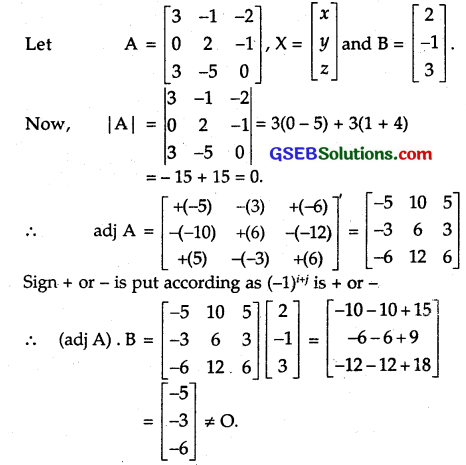∴ Given system equation is inconsistent.Question 6.
5x – y + 4z = 5
2x + 3y + 5z = 2
5x – 2y + 6z = – 1
Solution:
The given System of equation is
5x – y + 4z = 5
2x + 3y + 5z = 2
5x – 2y + 6z = – 1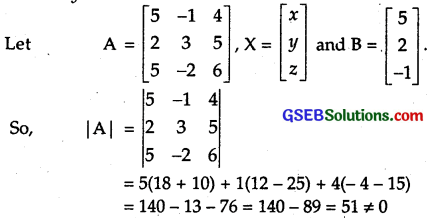⇒ The given System of equations is consistent.

Question 7.
5x + 2y = 4
7x + 3y = 5
Solution:
The System of equation is
5x + 2y = 4
7x + 3y = 5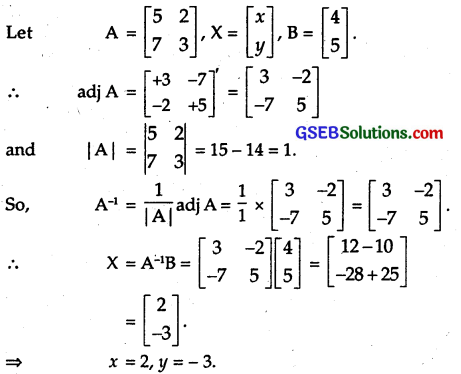Question 8.
2x – y = – 2
3x + 4y = 3
Solution:
The system of equations is
2x – y = – 2
3x + 4y = 3
This may be wirtten as AX = B.
or X = A-1B,Question 9.
4x – 3y = 3
3x – 5y = 7
Solution:
The system of equations is
4x – 3y = 3
3x – 5y = 7
This system of equations may be put as AX = B or X = A-1B,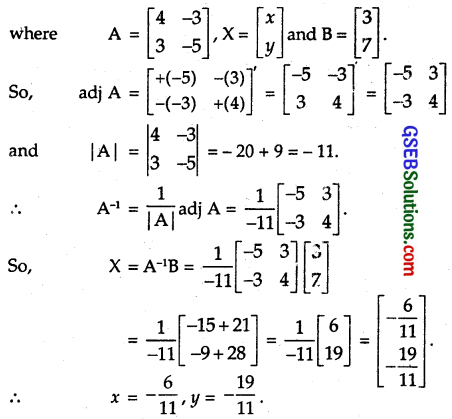Question 10.
5x + 2y = 3
3x + 2y = 5
Solution:
The system of equations is
5x + 2y = 3
3x + 2y = 5
This system of equations may be written as AX = B or X = A-1B,Question 11.
2x + y + z = 1
x – 2y – z = $$\frac { 3 }{ 2 }$$
3y – 5z = 9
Solution:
The given system of equations is
2x + y + z = 1
x – 2y – z = $$\frac { 3 }{ 2 }$$
3y – 5z = 9
This system of equations may be written as AX = B or X = A-1B,[+ or – signs are put according as ,(-i)i+i is +ve or -ve respectively. Minors of corresponding elements are written in brackets ()].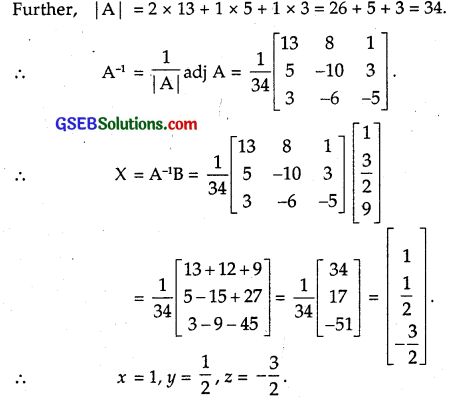Question 12.
x – y + z = 4
2x + y – 3z = 0
x + y + z = 2
Solution:
The given system of equations is
x – y + z = 4
2x + y – 3z = 0
x + y + z = 2
which may be written as AX = B or X = A-1B,
where A = $$\left[\begin{array}{ccc} 1 & -1 & 1 \\ 2 & 1 & -3 \\ 1 & 1 & 1 \end{array}\right]$$, X = $$\left[\begin{array}{l} x \\ y \\ z \end{array}\right]$$ and B = $$\left[\begin{array}{l} 4 \\ 0 \\ 2 \end{array}\right]$$
To find adj A, we calculate the cofactors of the elements of A.Question 13.
2x + + 3z = 5
x – 2y + z = – 4
3x – y – 2z = 3
Solution:
The given system of equations can be written as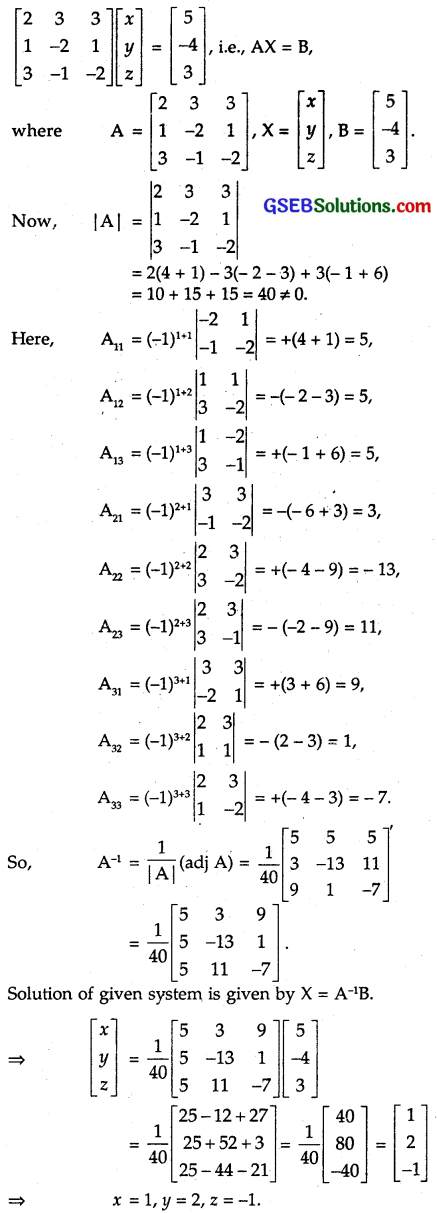Question 14.
x – y + 2z = 7
3x + 4y – 5z = – 5
2x – y + 3z = 12
Solution:
The given system of equation can be written as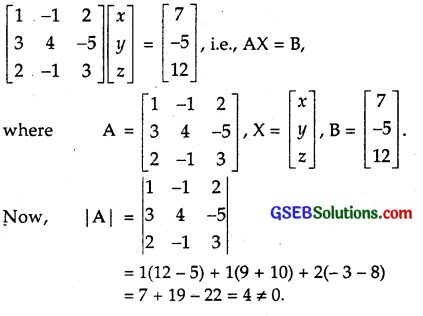∴ A-1 exists and hence the given equations have a unique solution.
Here,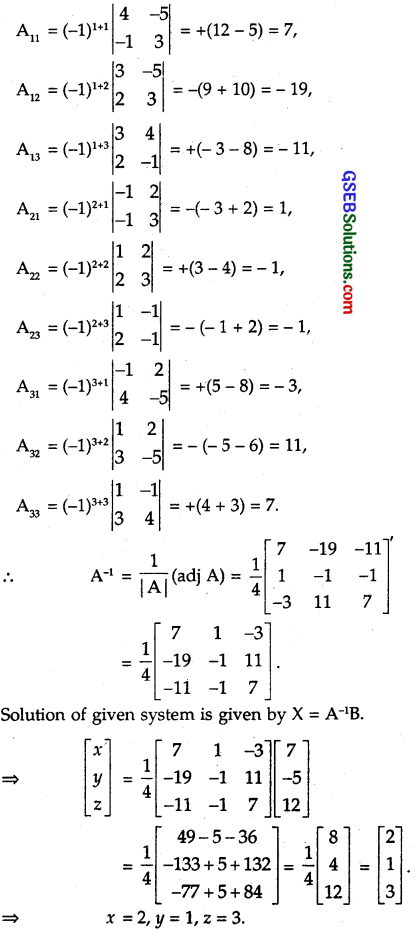Question 15.
If A = $$\left[\begin{array}{ccc} 2 & -3 & 5 \\ 3 & 2 & -4 \\ 1 & 1 & -2 \end{array}\right]$$, find A-1. Using A-1, solve the following system of linear equations:
2x – 3y + 5z = 11, 3x + 2y – 4z = – 5, x + y – 2z = – 3.
Solution:
The given system of equations can be written as
$$\left[\begin{array}{ccc} 2 & -3 & 5 \\ 3 & 2 & -4 \\ 1 & 1 & -2 \end{array}\right]$$$$\left[\begin{array}{l} x \\ y \\ z \end{array}\right]$$ = $$\left[\begin{array}{l} 11 \\ -5 \\ -3 \end{array}\right]$$, i.e., AX = B,
Now, |A| = $$\left|\begin{array}{ccc} 2 & -3 & 5 \\ 3 & 2 & -4 \\ 1 & 1 & -2 \end{array}\right|$$
= 2(- 4 + 4) + 3(- 6 + 6) + 5(3 – 2)
= 0 – 6 + 5 = – 1 ≠ 0.
∴ A-1 exists and hence the given equations have a unique solution.Question 16.
The cost of 4 kg onions, 3 kg wheat and 2kg rice is ₹ 60. The cost of 2 kg onions, 4 kg wheat and 6 kg rice ₹ 90. The cost of 6 kg onions, 2 kg wheat and 3 kg rice is 70. Find the cost of each item per kg by matrix method.
Solution:
Let the cost of ornons, wheat and rice per kg be ₹ x, ₹ y and ₹ z respectively.
The cost of 4 kg onions, 3 kg wheat and 2 kg rice = ₹ 60.
∴ 4x + 3y + 2z = 60. … (1)
The cost of 2 kg onions, 4 kg wheat and 6 kg rice = ₹ 90
∴ 2x + 4y + 6z = 90 … (2)
The cost of 6 kg onions, 2 kg wheat and 3 kg rice = ₹ 70
∴ 6x + 2y + 3z = 70 … (3)
The system of equations is
4x + 3y + 2z = 60
x + 2y + 3z = 45 [Dividing equation (2) by 2]
6x + 2y + 3z = 70,
which may be written as
AX = B or X = A-1B,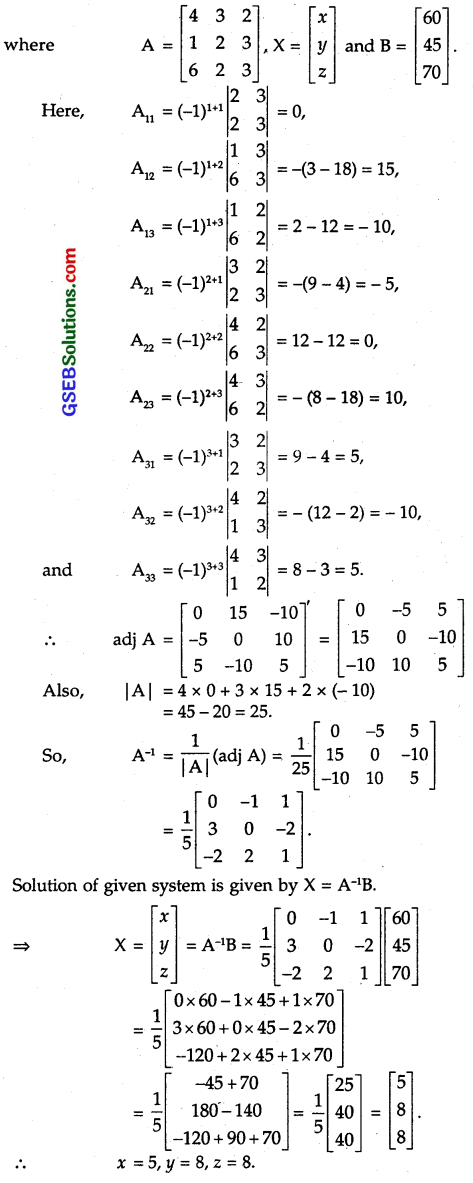⇒ The cost of onions, wheat and rice per kg are ₹ 5, ₹ 8, ₹ 8 respectively.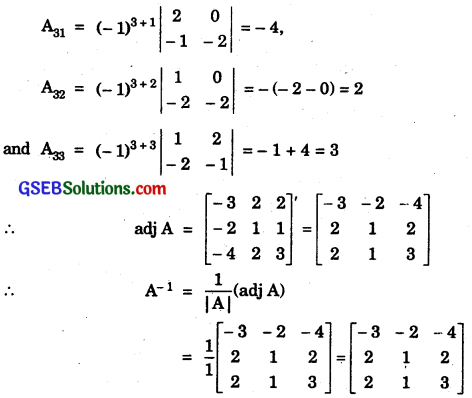Also, we have given equations as
x – 2y = 10
2x – y – z = 8
and – 2y + z = 7
or $$\left[\begin{array}{ccc} 1 & -2 & 0 \\ 2 & -1 & -1 \\ 0 & -2 & 1 \end{array}\right]$$$$\left[\begin{array}{l} x \\ y \\ z \end{array}\right]$$ = $$\left[\begin{array}{l} 10 \\ 8 \\ 7 \end{array}\right]$$
or CX = D,
where C = $$\left[\begin{array}{ccc} 1 & -2 & 0 \\ 2 & -1 & -1 \\ 0 & -2 & 1 \end{array}\right]$$, X = $$\left[\begin{array}{l} x \\ y \\ z \end{array}\right]$$
and D = $$\left[\begin{array}{l} 10 \\ 8 \\ 7 \end{array}\right]$$.
We know that (A’)-1 = (A-1)’.
∴ C’ = $$\left[\begin{array}{ccc} 1 & -2 & 0 \\ – 2 & -1 & -2 \\ 0 & – 1 & 1 \end{array}\right]$$